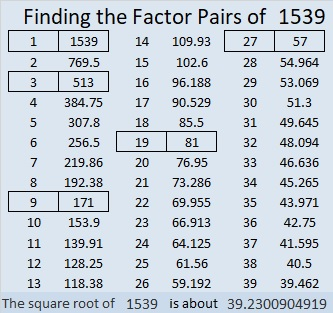# 1539 Mystery Puzzle for a Mystery Message

Contents

### Today’s Puzzle:

This is the third letter in my message to you. Have you figured out the complete message yet?

The puzzle itself is a mystery level puzzle. Will it be easy or difficult to do? You’ll have to try it to know the answer to that one!### Factors of 1539:

Adding up the non-nine digits we get 1 + 5 + 3 = 9, so 1539 is divisible by 9. In fact, it is divisible by 9 twice!

• 1539 is a composite number.
• Prime factorization: 1539 = 3 × 3 × 3 × 3 × 19, which can be written 1539 = 3⁴ × 19.
• 1539 has at least one exponent greater than 1 in its prime factorization so √1539 can be simplified. Taking the factor pair from the factor pair table below with the largest square number factor, we get √1539 = (√81)(√19) = 9√19.
• The exponents in the prime factorization are 4 and 1. Adding one to each exponent and multiplying we get (4 + 1)(1 + 1) = 5 × 2 = 10. Therefore 1539 has exactly 10 factors.
• The factors of 1539 are outlined with their factor pair partners in the graphic below.### More about the Number 1539:

1539 is the difference of two squares in five different ways:
770² – 769² = 1539,
258² – 255² = 1539,
90² – 81² = 1539,
50² – 31² = 1539, and
42² – 15² = 1539.

This site uses Akismet to reduce spam. Learn how your comment data is processed.